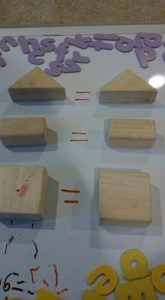# Teaching a Preschooler to Write Equations

We have done much hands-on work with my preschooler for learning to count and add. See: How I Taught My 3 Year Old to Count to 100.

When I went to teach him how to write out the equation, a level above this hands-on work, I took some unifix cubes, added, say, 1 cube and 2 cubes together, and then wrote out the equation on a white board on an easel that we have. My son totally gets how to add, but when he took his turn at writing out equations, without the cubes, he would usually write, “1 + 1 = 11.”

I tried showing him how 1 cube plus 2 cubes equals 3, then I would write it on the white board. It didn’t work. Once he wrote “1 + 2 = 3.” My heart jumped! Then he wrote “2 + 3 = 4.” Bummer.

This below activity resolved it. It’s just a slight difference from what I did, but a powerful one. I had read to put some number of objects in little bowls with a “+” sign in between them and an equal sign after, and another bowl. The objects and the equation would then be united: the objects being so close to the equation being written (as opposed to doing it then transferring to a distant place to write the equation).

I found the below to be even easier. I took our white board (a different one) and laid it on the floor. I then wrote “1” and put 1 unifix cube under it. Then “+” then another “1” with another cube under it, followed by “=”.  He totally got this. He picked up the cubes immediately, added them, and wrote the number “2.” He stayed with me for the next equations,To reinforce the idea of an “=” sign, that either side of the equation is the same, we also did this activity, where we matched shapes, not numbers together. After I did this, he went to our chalkboard and drew a triangle and wrote “=” and drew another triangle. I can only hope that this is planting the groundwork for the Law of Identity, that something is itself, a foundation of objectivity, in his mind : )I also showed him how to use a calculator. While doing it, I was saying “1 plus 1 equals 2,” as I punched it out on the calculator. But then I remembered Montessori’s advice that if you talk while giving a lesson, the child watches your lips. If you shut up and demonstrate, they watch your hands. So, I did. He was so enthralled with what result the calculator would give that he watched me as I used the calculator.

I did this yesterday, and today he came bursting into my room this morning to tell me, “Mom!! 10 plus 10 is 20!!!” Yes, that children do best with a lesson one day, the chance to sleep on it, then given a chance to execute it the NEXT day continues to be a tried and true paradigm.

He is now an equation writing, calculator using, unifix cube adding machine, without me even asking him to do these lessons. Set the groundwork, then let them practice, practice, practice – on their own initiative!